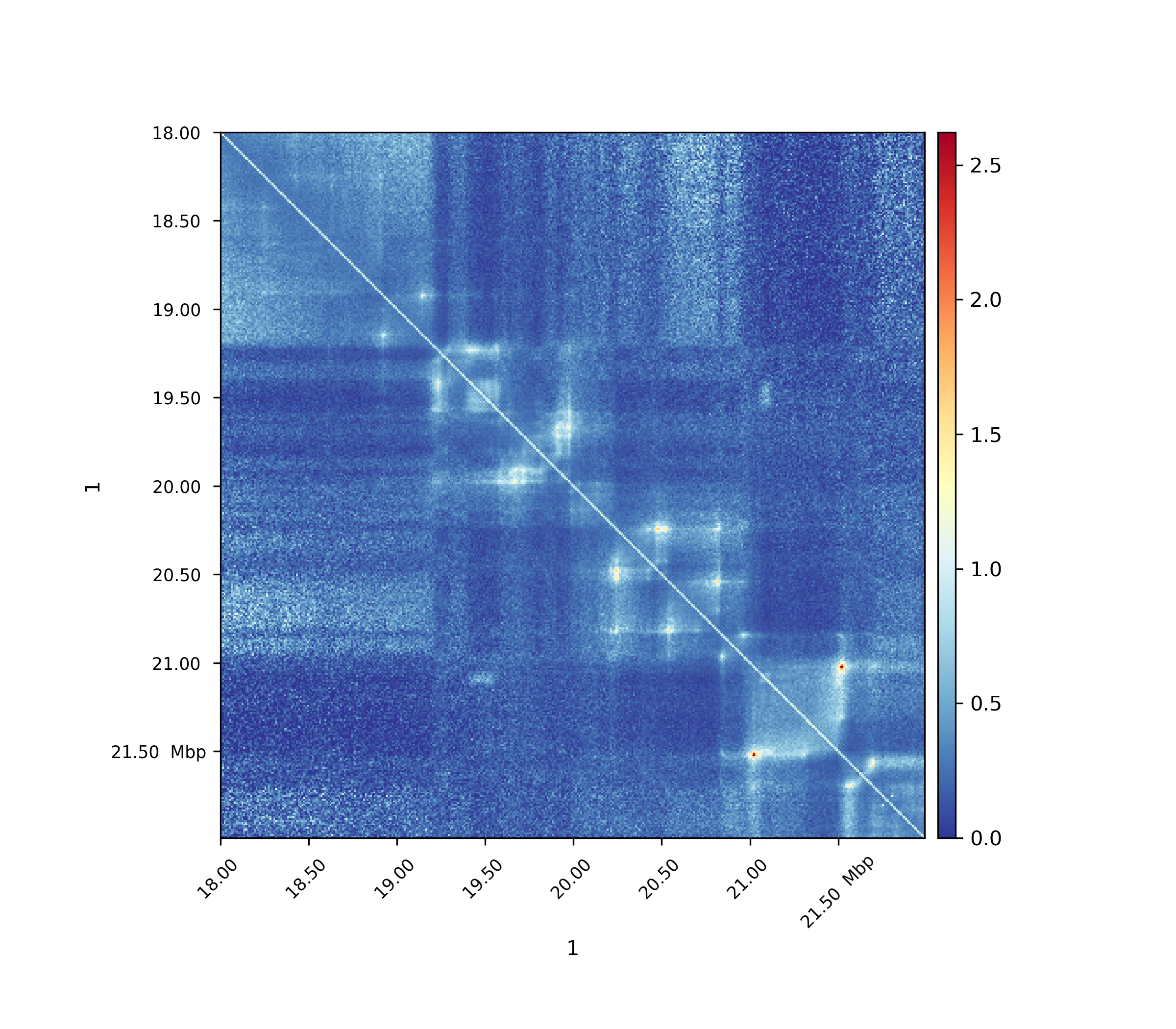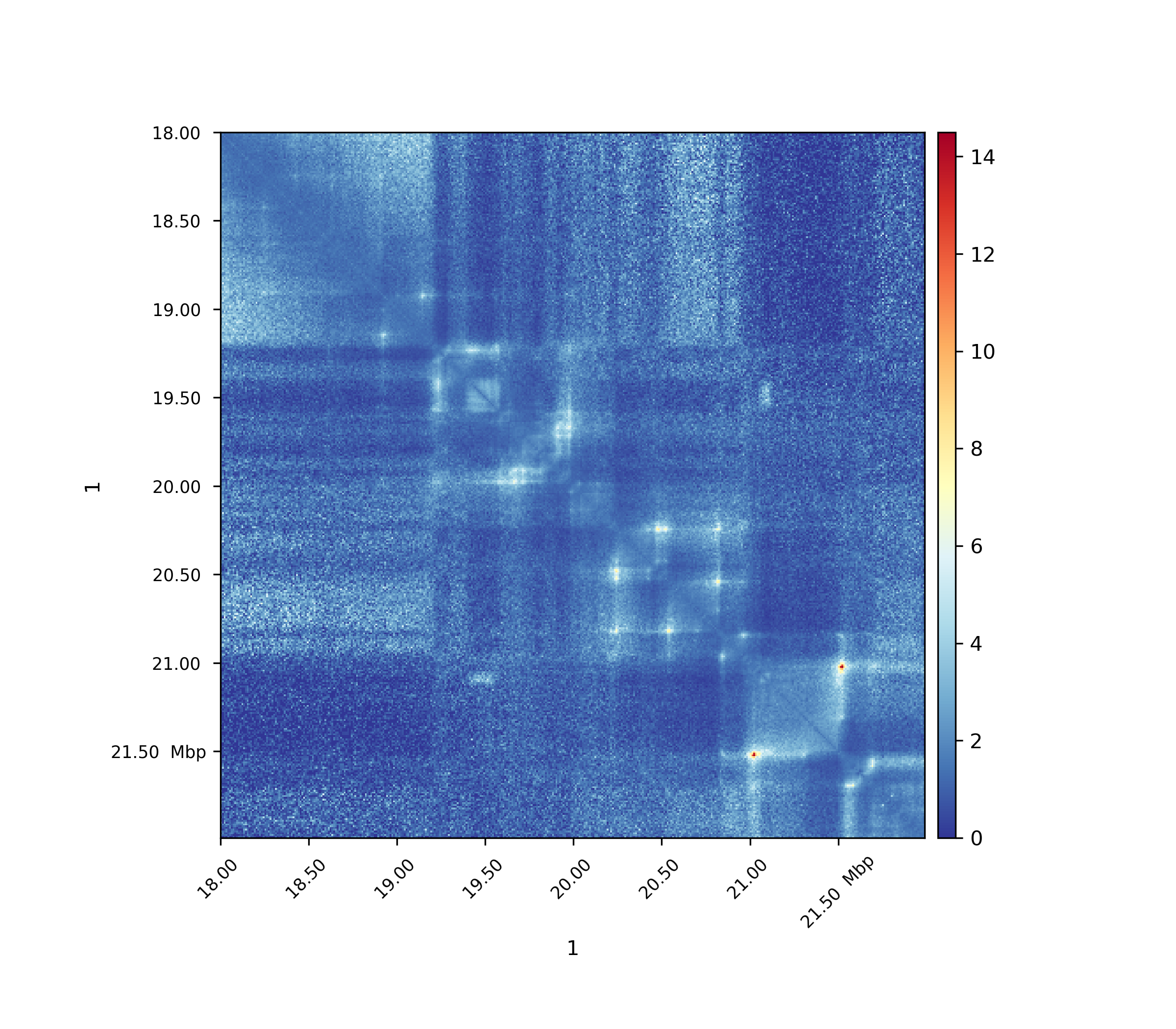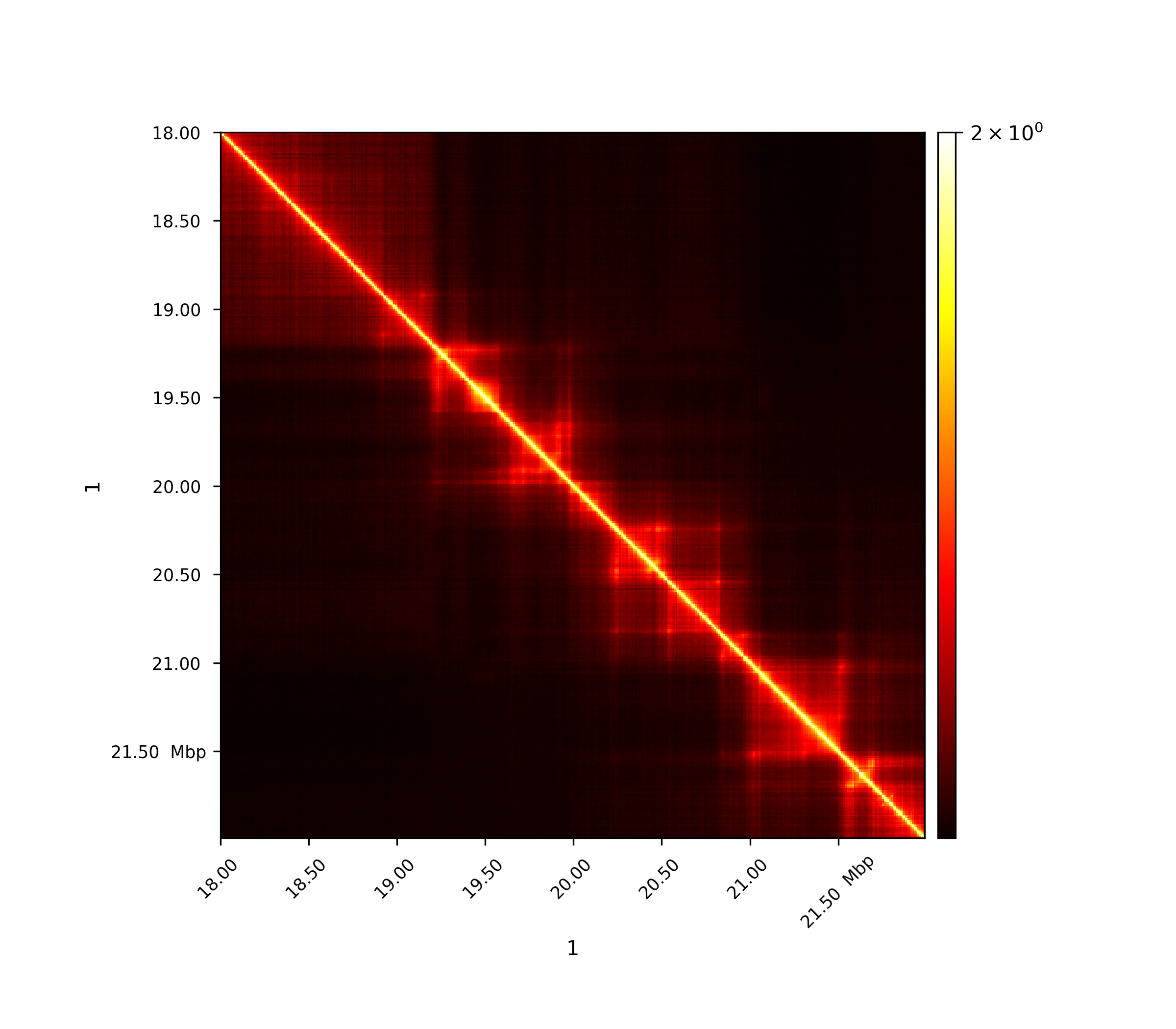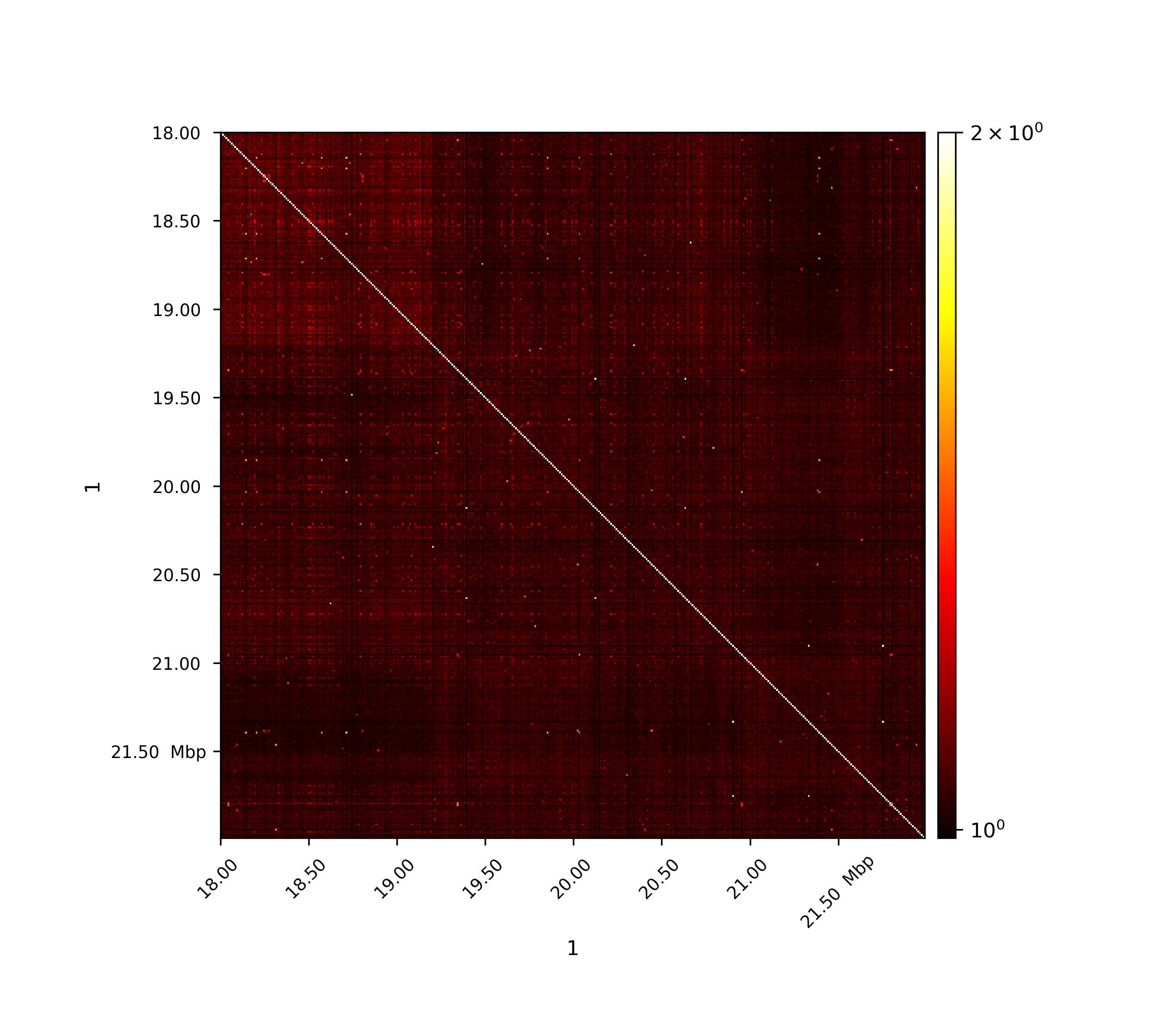# hicTransform¶

Converts the (interaction) matrix to different types of obs/exp, pearson or covariance matrix.

usage: hicTransform --matrix MATRIX --outFileName OUTFILENAME
[--method {obs_exp,obs_exp_lieberman,obs_exp_non_zero,pearson,covariance}]
[--ligation_factor]
[--chromosomes CHROMOSOMES [CHROMOSOMES ...]]
[--perChromosome] [--help] [--version]


## Required arguments¶

--matrix, -m

input file. The computation is done per chromosome.

--outFileName, -o

File name to save the exported matrix.

## Optional arguments¶

--method, -me

Possible choices: obs_exp, obs_exp_lieberman, obs_exp_non_zero, pearson, covariance

Transformation methods to use for input matrix. Transformation is computed per chromosome.obs_exp computes the expected matrix as the sum per genomic distance j divided by maximal possible contacts: sum(diagonal(j) / number of elements in diagonal(j) obs_exp_lieberman computes the expected matrix as the sum per genomic distance j divided by the : sum(diagonal(j) / (length of chromosome - j))obs_exp_non_zero computes the expected matrix as the sum per genomic distance j divided by sum of non-zero contacts: sum(diagonal(j) / number of non-zero elements in diagonal(j)Optionally, –ligation_factor can be used for this method as has been used by HOMER software. If –ligation_factor, then exp_i,j = exp_i,j * sum(row(i)) * sum(row(j)) / sum(matrix)pearson computes the Pearson correlation of the input matrix: Pearson_i,j = C_i,j / sqrt(C_i,i * C_j,j) and C is the covariance matrixcovariance computes the Covariance of the input matrix: Cov_i,j = E[M_i, M_j] - my_i * my_j where M is the input matrix and my the mean (Default: “obs_exp”).

Default: “obs_exp”

--ligation_factor

Setting this flag, multiplies a scaling factor to each entry of the expected matrix to take care of the proximity ligation as has been explained in Homer software. This flag is only affective with obs_exp_non_zero method and will be ignored if any other obs/exp method is chosen.

Default: False

--chromosomes

List of chromosomes to be included in the computation.

--perChromosome, -pc

Each chromosome is processed individually, inter-chromosomal interactions are ignored. Option not valid for obs_exp_lieberman.

Default: False

--version

show program’s version number and exit

## Background¶

hicTransform transforms a given input matrix into a new matrix using one of the following methods:

• obs_exp

• obs_exp_lieberman

• obs_exp_non_zero

• pearson

• covariance

All expected values are computed per genomic distances.

## Usage¶

\$ hicTransform -m matrix.cool --method obs_exp -o obs_exp.cool
`

For all images data from Rao 2014 was used.

## Observed / Expected¶

All values, including non-zero values, are used to compute the expected values per genomic distance.

$exp_{i,j} = \frac{ \sum diagonal(|i-j|) }{|diagonal(|i-j|)|}$## Observed / Expected lieberman¶

The expected matrix is computed in the way as Lieberman-Aiden used it in the 2009 publication. It is quite similar to the obs/exp matrix computation.

$exp_{i,j} = \frac{ \sum diagonal(|i-j|) } {(length\ of\ chromosome\ - |i-j|))}$## Observed / Expected non zero¶

Only non-zero values are used to compute the expected values per genomic distance, i.e. only non-zero values are taken into account for the denominator.

$exp_{i,j} = \frac{ \sum diagonal(i-j) }{ number\ of\ non-zero\ elements\ in\ diagonal(|i-j|)}$By adding the –ligation_factor flag, the expected matrix can be re-scaled in the same way as has been done by Homer software when computing observed/expected matrices with the option ‘-norm’.

$exp_{i,j} = exp_{i,j} * \sum row(j) * \sum row(i) }{ \sum matrix }$## Pearson correlation matrix¶

$Pearson_{i,j} = \frac {C_{i,j} }{ \sqrt{C_{i,i} * C_{j,j} }}$

C is the covariance matrixThe first image shows the Pearson correlation on the original interaction matrix, the second one shows the Person correlation matrix on an observed/expected matrix. A consecutive computation like this is used in the A/B compartment computation.

## Covariance matrix¶

$Cov_{i,j} = E[M_i, M_j] - \mu_i * \mu_j$

where M is the input matrix and $$\mu$$ the mean.